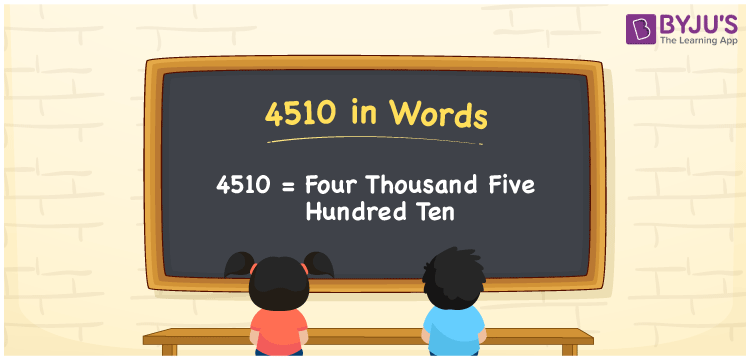# 4510 in Words

The word form of the number 4510 is Four Thousand Five Hundred Ten. For instance, Rahul purchased a weighing machine for Rs. 4510, then you can say, “Rahul purchased a weighing machine for Rupees Four Thousand Five Hundred Ten”. 4510 is a cardinal number as it indicates a certain amount. In this article, we will learn the simple tricks of writing the numerical name for the number 4510.

 4510 in Words Four Thousand Five Hundred Ten Four Thousand Five Hundred Ten in numerical form 4510

## 4510 in English Words

We usually, write the numbers in words using the letters of the English alphabet. Hence, we can write and spell the number 4510 in English as Four Thousand Five Hundred Ten.## How to Write 4510 in Words?

The table mentioned below depicts the place value chart for the number 4510 with 4 columns as it is a four-digit number.

 Thousands Hundreds Tens Ones 4 5 1 0

Hence, we can write the expanded form as:

4 x Thousand + 5 x Hundred + 1 x Ten + 0 x One

= 4 x 1000 + 5 x 100 + 1 x 10 + 0 x 1

= 4000 + 500 + 10 + 0

= 4000 + 500 + 10

= 4510

= Four Thousand Five Hundred Ten

Therefore, 4510 in words is written as Four Thousand Five Hundred Ten

Interesting way of writing 4510 in words

4 = Four

45 = Forty-Five

451 = Four Hundred Fifty-One

4510 = Four Thousand Five Hundred Ten

Thus, the word form of the number 4510 is Four Thousand Five Hundred Ten

4510 is a natural number that precedes 4511 and succeeds 4509

• 4510 in words – Four Thousand Five Hundred Ten
• Is 4510 an odd number? – No
• Is 4510 an even number? – Yes
• Is 4510 a perfect square number? – No
• Is 4510 a perfect cube number? – No
• Is 4510 a prime number? – No
• Is 4510 a composite number? – Yes

## Frequently Asked Questions on 4510 in Words

Q1

### How do you write 4510 in words?

We write 4510 in words as Four Thousand Five Hundred Ten.
Q2

### Simplify 3000 + 1510, and express in words.

Simplifying 3000 + 1510, we get 4510. Hence, the number 4510 in words is Four Thousand Five Hundred Ten.
Q3

### 4510 is a composite number. True or False.

True, the number 4510 is a composite number.